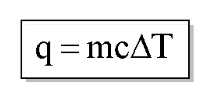# Problem: A sample of 0.562 g of carbon is burned in oxygen in a bomb calorimeter, producing carbon dioxide. Assume both the reactants and products are under standard state conditions, and that the heat released is directly proportional to the enthalpy of combustion of graphite. The temperature of the calorimeter increases from 26.74°C to 27.93°C. What is the heat capacity of the calorimeter and its contents?

###### FREE Expert Solution
• Amount of heat can be calculated using the calorimeter equation:• Where q as heat transferred (unknown) ; m is equal to the mass of the calorimeter; c as specific heat of calorimeter (unknown) and Δ T as change in temperature
• Q will be calculated first followed by the c of the calorimeter. We will be using the calorimeter equation and the constant value of the standard molar enthalpy.
• mass of bomb calorimeter can be considered as constant where equation be simplified as:
100% (486 ratings)###### Problem Details

A sample of 0.562 g of carbon is burned in oxygen in a bomb calorimeter, producing carbon dioxide. Assume both the reactants and products are under standard state conditions, and that the heat released is directly proportional to the enthalpy of combustion of graphite. The temperature of the calorimeter increases from 26.74°C to 27.93°C. What is the heat capacity of the calorimeter and its contents?